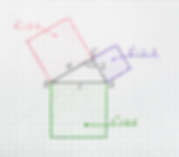## ALGEBRA

Growing IQ first introduces students to arithmetic in isolation, building a foundation of what each operation means and how to utilize them with accuracy.

As knowledge and familiarity increase, students begin to identify relationships between operations. This transition from arithmetic to algebraic thinking allows for a true understanding of the properties of operations.## 5 Categories of Algebra## ARITHMETIC

Arithmetic deals with the properties and manipulations of numbers in calculations.  Mastering these skills sets the building blocks for a child's entire mathematical academic career.## Discovering the properties of arithmetic develops an understanding of the way in which numbers behave when operations are used on them.  The ability to apply, recognize, and understand number properties are foundational to ongoing success in mathematics.## SEQUENCES

Analyzing the regularity of patterns allows for the identification of how things are related and the ability to derive formulas that explain those associations.  This type of thinking is fundamental to higher mathematical operations and necessary in virtually all computer applications.## Exponents are a shorthand mathematical notation for multiplying a number by itself several times.  The use of exponents becomes more frequent as the numbers being used in computation become larger, as formulas become more complicated, and as measurement is explored.## VARIABLES

Variables are a symbolic representation of unknown values.  They are often used in algebra to represent terms in equations, as well as to make generalizations about specific situations.  Almost all mathematic theorems are proven through the use of variables.